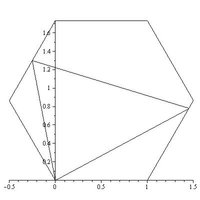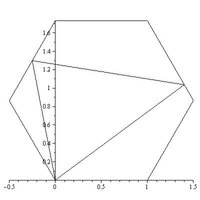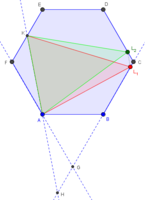#### Atti0626

##### New member
There is a regular hexagon ABCDEF. Let the halving point of EF be K. Find point L on side AB, BC or CD, where it is true that the area of the AKL triangle equals 2/5 of the area of the hexagon.

I let the side of the hexagon be 1, and calculated that the AK segment's length is sqrt(7)/2, and the height of the triangle needs to be 12×sqrt(3)/5×sqrt(7).

I also tried out what happens if I let L be B, C, D, or the halving points of these sides.

I think my approach to this problem was wrong, but I couldn't figure out any other way to do it.

Any help or idea is appreciated.

#### ksdhart2

##### Senior Member
I'd start by constructing a unit hexagon such that the vertices are placed at $$\displaystyle \sin\left(\frac{k\pi}{3}\right), \cos\left(\frac{k\pi}{3}\right)$$ for $$k = 0, 1, \cdots, 5$$. By doing this, we can see that $$A = (0,1)$$ and $$K$$ being the midpoint of $$\overline{EF}$$ means that $$\displaystyle K = \left( \frac{\sqrt{3}}{2}, 0 \right)$$. Then we can use the formula for the area of a triangle given three vertices:

$$\displaystyle \text{Area} \: = \: \biggr| \frac{A.x\left(K.y-L.y\right)+K.x\left(L.y-A.y\right)+L.x\left(A.y-K.y\right)}{2} \biggl|$$

The area of a unit hexagon is $$\displaystyle \frac{3\sqrt{3}}{2}$$, so that means we want $$\displaystyle \triangle AKL$$ to have an area of $$\displaystyle \frac{3\sqrt{3}}{5}$$. Of the three options given in the problem text, deciding that $$L$$ should lie on $$\overline{BC}$$ is the easiest, since that fixes the x-coordinate in place, leaving us just one variable to solve for. Plugging in all of the known information and the desired area, we get an equation to solve:

$$\displaystyle \frac{3\sqrt{3}}{5} = \: \biggr| \frac{-\frac{\sqrt{3}}{2}\left(L.y-1\right)+\frac{\sqrt{3}}{2}}{2} \biggl|$$

...although now that I've written this all down, I'm not actually sure it solves the problem at hand, because it makes quite a few (unjustified) assumptions about the placement of the vertices and the area of the hexagon. Presumably, they want you to arrive at some revelation regarding how $$L$$ might be positioned relative to $$B$$ and $$C$$, but I'm not quite seeing how just yet. In any case, I'll leave all of this as it is, in the hopes that my partial workings at least get you thinking in the right direction and then maybe you can take it the rest of the way. Good luck!

#### Dr.Peterson

##### Elite Member
I'd start by working out the areas if L is located at B, C, or D, as you said, to decide which side L must be one. (I don't think the midpoints are needed.) What did you find?

My approach to finding areas is to first consider the areas of pieces formed by various diagonals that together divide it into equal parts; for example, the area of triangle AEF is 1/6 (of the whole hexagon). Therefore triangle AKF is half of that. Then I suppose that L is x (fraction) of the way from vertex _ to vertex _, and use proportions to find areas of the parts constituting AKL, or the parts outside it. It's a bit of work, but might be nicer than ksdhart's analytical approach. (I did get an answer, but don't yet trust it.)

Your approach looks doable; one way to find the altitude to L would be to do that for the vertices on either side of it and use proportions.

#### Atti0626

##### New member
Thank you for your responses! I tried Dr. Peterson's method, and I got that L is on side DC, 13/20 units away from D. Did you get this as your answer too?

#### Atti0626

##### New member
I noticed that I messed up something, and my new answer is that it is on the AB side, 1/40 units away from B.

#### Dr.Peterson

##### Elite Member
I found two solutions, one on BC and one on CD.

What areas do you get for L = B, C, D, and E?

LCKurtz's area is far less than 2/5, and closer to 1/5 (of the area of the hexagon). None of the other answers match either. (Mine are verified in GeoGebra. But they could still be wrong -- I'm recovering from a visit to the dentist and may not be thinking clearly.)

#### Atti0626

##### New member
Okay, I think I got it right this time.
On AB, it is impossible.
On BC, it is 0.9 from B.
On CD, it is 0.8 from D.
Do those match your two solutions?

#### LCKurtz

##### Full Member
Dang! I hate not being able to edit posts. You're right, I found an error in my work. I will post again later today after I sort it out...

#### LCKurtz

##### Full Member
Naturally, I can't edit message #8 either. A real annoyance. Anyway, after correcting my syntax in Maple I get the following two answers. The first one has L $$\displaystyle \frac 9 {10}$$ the way from B to C:The second one has L $$\displaystyle \frac 1 5$$ the way from C to D:They look better and hopefully are correct.

#### Dr.Peterson

##### Elite Member
Yes, that agrees with my calculations.

Here is my check, with some additional points used in my calculation:Note that CL1 : CL2 = FK : FA = 1:2, which agrees with your (and my) numbers.

One way to obtain the exact answer with minimal work is to find area HKC (taking the area of the hexagon as a unit) and determine what HKL1 has to be, then find CL1 by a simple proportion. This is a lot easier than my original way.

#### Atti0626

##### New member
Thank you all for your help! In the end, I did it by calling one part of the side x, and the other 1-x, dividing the hexagon into triangled, and doing the calculations from there.

#### gandrew

##### New member
Yes, that agrees with my calculations.

Here is my check, with some additional points used in my calculation:

View attachment 12480

Note that CL1 : CL2 = FK : FA = 1:2, which agrees with your (and my) numbers.

One way to obtain the exact answer with minimal work is to find area HKC (taking the area of the hexagon as a unit) and determine what HKL1 has to be, then find CL1 by a simple proportion. This is a lot easier than my original way.
oh now i get what the problem was. i was just solving it for practice